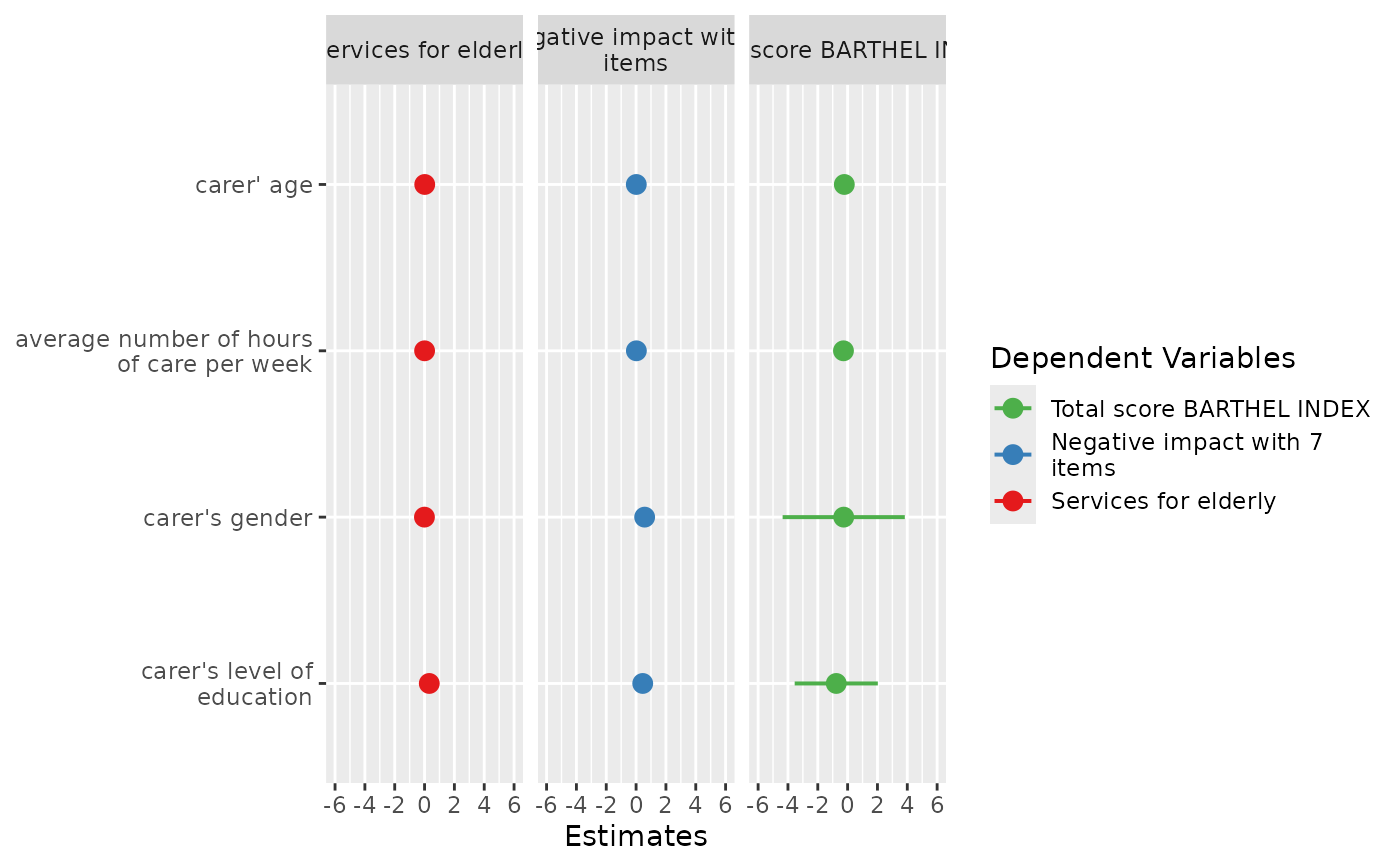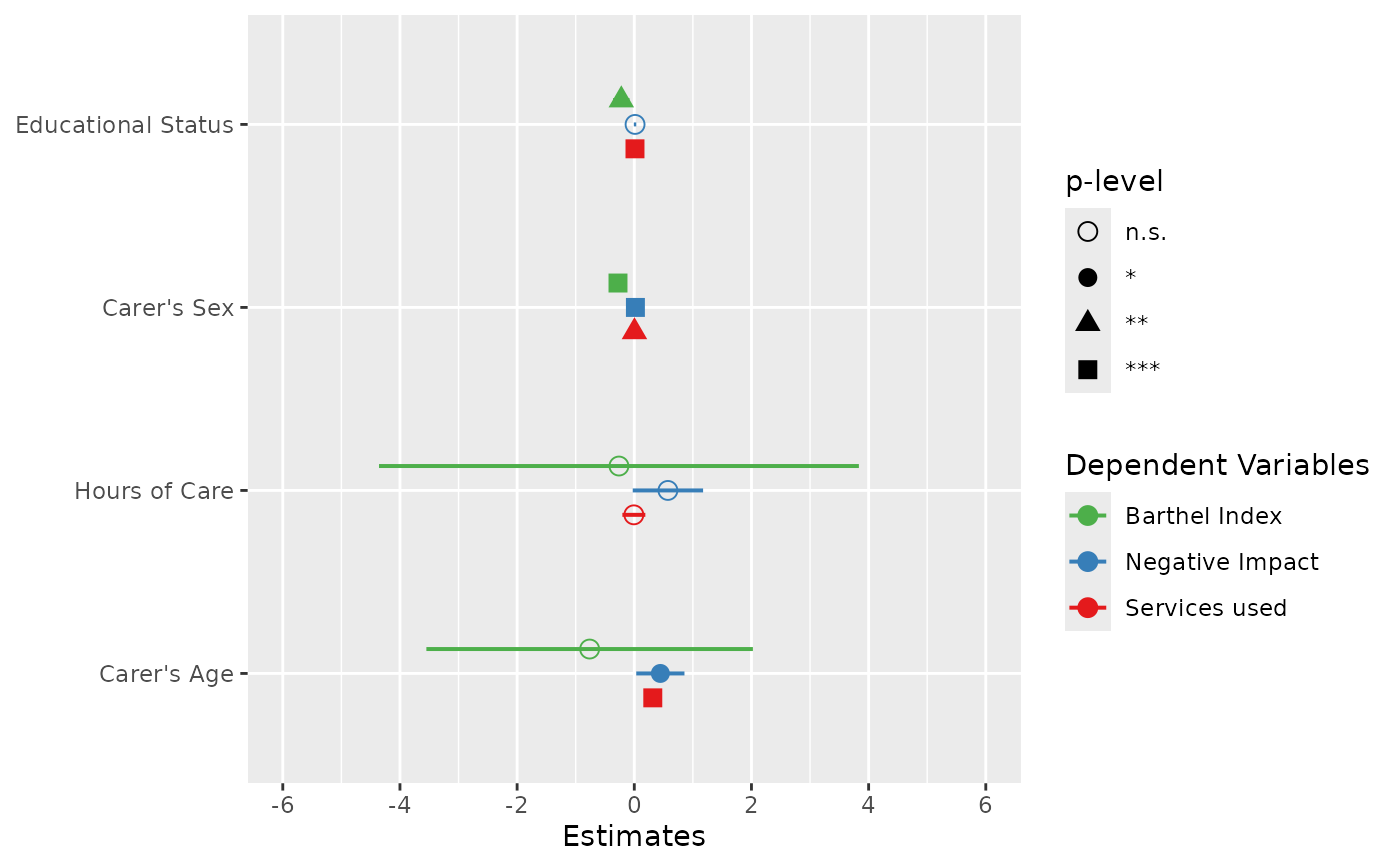Plot and compare regression coefficients with confidence intervals of multiple regression models in one plot.

plot_models(
...,
transform = NULL,
std.est = NULL,
rm.terms = NULL,
title = NULL,
m.labels = NULL,
legend.title = "Dependent Variables",
legend.pval.title = "p-level",
axis.labels = NULL,
axis.title = NULL,
axis.lim = NULL,
wrap.title = 50,
wrap.labels = 25,
wrap.legend.title = 20,
grid.breaks = NULL,
dot.size = 3,
line.size = NULL,
value.size = NULL,
spacing = 0.4,
colors = "Set1",
show.values = FALSE,
show.legend = TRUE,
show.intercept = FALSE,
show.p = TRUE,
p.shape = FALSE,
p.threshold = c(0.05, 0.01, 0.001),
ci.lvl = 0.95,
robust = FALSE,
vcov.fun = NULL,
vcov.type = c("HC3", "const", "HC", "HC0", "HC1", "HC2", "HC4", "HC4m", "HC5"),
vcov.args = NULL,
vline.color = NULL,
digits = 2,
grid = FALSE,
auto.label = TRUE,
prefix.labels = c("none", "varname", "label")
)

## Arguments

...

One or more regression models, including glm's or mixed models. May also be a list with fitted models. See 'Examples'.

transform

A character vector, naming a function that will be applied on estimates and confidence intervals. By default, transform will automatically use "exp" as transformation for applicable classes of model (e.g. logistic or poisson regression). Estimates of linear models remain untransformed. Use NULL if you want the raw, non-transformed estimates.

std.est

Choose whether standardized coefficients should be used for plotting. Default is no standardization (std.est = NULL). May be "std" for standardized beta values or "std2", where standardization is done by rescaling estimates by dividing them by two sd.

rm.terms

Character vector with names that indicate which terms should be removed from the plot. Counterpart to terms. rm.terms = "t_name" would remove the term t_name. Default is NULL, i.e. all terms are used. For factors, levels that should be removed from the plot need to be explicitely indicated in square brackets, and match the model's coefficient names, e.g. rm.terms = "t_name [2,3]" would remove the terms "t_name2" and "t_name3" (assuming that the variable t_name was categorical and has at least the factor levels 2 and 3). Another example for the iris dataset would be rm.terms = "Species [versicolor,virginica]". Note that the rm.terms-argument does not apply to Marginal Effects plots.

title

Character vector, used as plot title. By default, response_labels is called to retrieve the label of the dependent variable, which will be used as title. Use title = "" to remove title.

m.labels

Character vector, used to indicate the different models in the plot's legend. If not specified, the labels of the dependent variables for each model are used.

legend.title

Character vector, used as legend title for plots that have a legend.

legend.pval.title

Character vector, used as title of the plot legend that indicates the p-values. Default is "p-level". Only applies if p.shape = TRUE.

axis.labels

Character vector with labels for the model terms, used as axis labels. By default, term_labels is called to retrieve the labels of the coefficients, which will be used as axis labels. Use axis.labels = "" or auto.label = FALSE to use the variable names as labels instead. If axis.labels is a named vector, axis labels (by default, the names of the model's coefficients) will be matched with the names of axis.label. This ensures that labels always match the related axis value, no matter in which way axis labels are sorted.

axis.title

Character vector of length one or two (depending on the plot function and type), used as title(s) for the x and y axis. If not specified, a default labelling is chosen. Note: Some plot types may not support this argument sufficiently. In such cases, use the returned ggplot-object and add axis titles manually with labs. Use axis.title = "" to remove axis titles.

axis.lim

Numeric vector of length 2, defining the range of the plot axis. Depending on plot-type, may effect either x- or y-axis. For Marginal Effects plots, axis.lim may also be a list of two vectors of length 2, defining axis limits for both the x and y axis.

wrap.title

Numeric, determines how many chars of the plot title are displayed in one line and when a line break is inserted.

wrap.labels

Numeric, determines how many chars of the value, variable or axis labels are displayed in one line and when a line break is inserted.

wrap.legend.title

numeric, determines how many chars of the legend's title are displayed in one line and when a line break is inserted.

grid.breaks

Numeric value or vector; if grid.breaks is a single value, sets the distance between breaks for the axis at every grid.breaks'th position, where a major grid line is plotted. If grid.breaks is a vector, values will be used to define the axis positions of the major grid lines.

dot.size

Numeric, size of the dots that indicate the point estimates.

line.size

Numeric, size of the lines that indicate the error bars.

value.size

Numeric, indicates the size of value labels. Can be used for all plot types where the argument show.values is applicable, e.g. value.size = 4.

spacing

Numeric, spacing between the dots and error bars of the plotted fitted models. Default is 0.3.

colors

May be a character vector of color values in hex-format, valid color value names (see demo("colors")) or a name of a pre-defined color palette. Following options are valid for the colors argument:

• If not specified, a default color brewer palette will be used, which is suitable for the plot style.

• If "gs", a greyscale will be used.

• If "bw", and plot-type is a line-plot, the plot is black/white and uses different line types to distinguish groups (see this package-vignette).

• If colors is any valid color brewer palette name, the related palette will be used. Use RColorBrewer::display.brewer.all() to view all available palette names.

• There are some pre-defined color palettes in this package, see sjPlot-themes for details.

• Else specify own color values or names as vector (e.g. colors = "#00ff00" or colors = c("firebrick", "blue")).

show.values

Logical, whether values should be plotted or not.

show.legend

For Marginal Effects plots, shows or hides the legend.

show.intercept

Logical, if TRUE, the intercept of the fitted model is also plotted. Default is FALSE. If transform = "exp", please note that due to exponential transformation of estimates, the intercept in some cases is non-finite and the plot can not be created.

show.p

Logical, adds asterisks that indicate the significance level of estimates to the value labels.

p.shape

Logical, if TRUE, significant levels are distinguished by different point shapes and a related legend is plotted. Default is FALSE.

p.threshold

Numeric vector of length 3, indicating the treshold for annotating p-values with asterisks. Only applies if p.style = "asterisk".

Character vector, if not NULL, indicates the method to adjust p-values. See p.adjust for details.

ci.lvl

Numeric, the level of the confidence intervals (error bars). Use ci.lvl = NA to remove error bars. For stanreg-models, ci.lvl defines the (outer) probability for the credible interval that is plotted (see ci). By default, stanreg-models are printed with two intervals: the "inner" interval, which defaults to the 50%-CI; and the "outer" interval, which defaults to the 89%-CI. ci.lvl affects only the outer interval in such cases. See prob.inner and prob.outer under the ...-argument for more details.

robust

Deprecated. Please use vcov.fun directly to specify the estimation of the variance-covariance matrix.

vcov.fun

Variance-covariance matrix used to compute uncertainty estimates (e.g., for robust standard errors). This argument accepts a covariance matrix, a function which returns a covariance matrix, or a string which identifies the function to be used to compute the covariance matrix. See model_parameters().

vcov.type

Deprecated. The type-argument is now included in vcov.args.

vcov.args

List of arguments to be passed to the function identified by the vcov.fun argument. This function is typically supplied by the sandwich or clubSandwich packages. Please refer to their documentation (e.g., ?sandwich::vcovHAC) to see the list of available arguments.

vline.color

Color of the vertical "zero effect" line. Default color is inherited from the current theme.

digits

Numeric, amount of digits after decimal point when rounding estimates or values.

grid

Logical, if TRUE, multiple plots are plotted as grid layout.

auto.label

Logical, if TRUE (the default), and data is labelled, term_labels is called to retrieve the labels of the coefficients, which will be used as predictor labels. If data is not labelled, format_parameters() is used to create pretty labels. If auto.label = FALSE, original variable names and value labels (factor levels) are used.

prefix.labels

Indicates whether the value labels of categorical variables should be prefixed, e.g. with the variable name or variable label. See argument prefix in term_labels for details.

A ggplot-object.

## Examples

data(efc)

# fit three models
fit1 <- lm(barthtot ~ c160age + c12hour + c161sex + c172code, data = efc)
fit2 <- lm(neg_c_7 ~ c160age + c12hour + c161sex + c172code, data = efc)
fit3 <- lm(tot_sc_e ~ c160age + c12hour + c161sex + c172code, data = efc)

# plot multiple models
plot_models(fit1, fit2, fit3, grid = TRUE)# plot multiple models with legend labels and
# point shapes instead of value labels
plot_models(
fit1, fit2, fit3,
axis.labels = c(
"Carer's Age", "Hours of Care", "Carer's Sex", "Educational Status"
),
m.labels = c("Barthel Index", "Negative Impact", "Services used"),
show.values = FALSE, show.p = FALSE, p.shape = TRUE
)if (FALSE) {
# plot multiple models from nested lists argument
all.models <- list()
all.models[] <- fit1
all.models[] <- fit2
all.models[] <- fit3

plot_models(all.models)

# plot multiple models with different predictors (stepwise inclusion),
# standardized estimates
fit1 <- lm(mpg ~ wt + cyl + disp + gear, data = mtcars)
fit2 <- update(fit1, . ~ . + hp)
fit3 <- update(fit2, . ~ . + am)

plot_models(fit1, fit2, fit3, std.est = "std2")
}